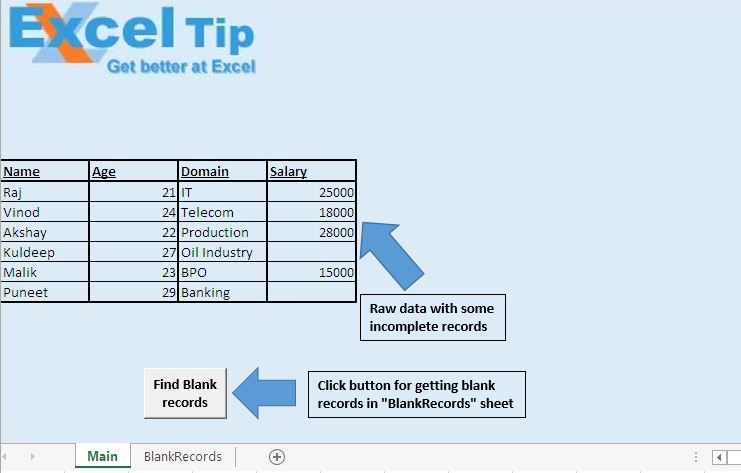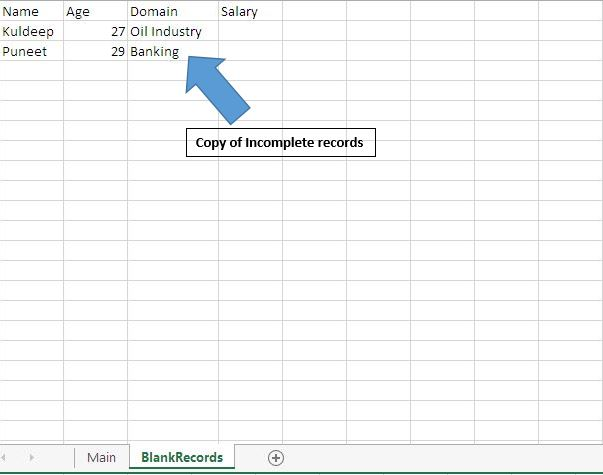# Copy incomplete data to a new sheet using VBA in Microsoft Excel

In this article, we will create a macro to copy incomplete data to a new sheet.

Raw data for this article consists of salary data, which includes some incomplete records.We want to copy the records of those employees whose salary details are missing. Clicking the button will move incomplete records to “BlankRecords” sheet.Logic explanation

In this article, we have created “CopyEmptys” macro to copy missing records to “BlankRecords” sheet. It checks the Salary column for blank records. If a blank record is encountered, then it copies that record to “BlankRecords” sheet.

Code explanation

intRowL = Cells(Rows.Count, 1).End(xlUp).Row

Above code is used to get row number of last cell.

IsEmpty(Cells(intRow, 4))

Above code is used to check whether mentioned salary is empty.

.Range(.Cells(intRowT, 1), .Cells(intRowT, 3)).Value = Range(Cells(intRow, 1), Cells(intRow, 3)).Value

Above code is used to have a copy of missing record from the main sheet to “BlankRecord” sheet.

```Option Explicit

Sub CopyEmptys()

'Declaring variables
Dim intRow As Integer, intRowL As Integer, intRowT As Integer

'Getting row number of last cell
intRowL = Cells(Rows.Count, 1).End(xlUp).Row

'Looping from 10th row to last cell
For intRow = 10 To intRowL

'Checking the fourth column whether it is empty
If IsEmpty(Cells(intRow, 4)) Then
With Worksheets(2)
'Getting row number of row next to last row
intRowT = .Cells(.Rows.Count, 1).End(xlUp).Row + 1

'Inserting data to "BlankRecords" sheet
.Range(.Cells(intRowT, 1), .Cells(intRowT, 3)).Value = _
Range(Cells(intRow, 1), Cells(intRow, 3)).Value
End With
End If
Next intRow

End Sub
```